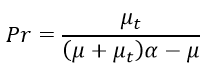# Prandtl-K Macro, UDF, Prandtl number CFD Simulation

\$135.00 Student Discount

• The problem numerically simulates the turbulence model using ANSYS Fluent software.
• We design the 2-D model with the Design Modeler software.
• We mesh the model with ANSYS Meshing software, and the element number equals 97,972.
• We use the User-Defined Function (UDF) to define the Prandtl number.
• We use the Prandtl_K Macro for UDF.
Click on Add To Cart and obtain the Geometry file, Mesh file, and a Comprehensive ANSYS Fluent Training Video. By the way, You can pay in installments through Klarna, Afterpay (Clearpay), and Affirm.

To Order Your Project or benefit from a CFD consultation, contact our experts via email ([email protected]), online support tab, or WhatsApp at +44 7443 197273.

There are some Free Products to check our service quality.

If you want the training video in another language instead of English, ask it via [email protected] after you buy the product.

## Description

In this project, we performed a numerical simulation using ANSYS Fluent software’s User-Defined Function (UDF). For this CFD product, we used Prandtl_K Macro to write UDF programming. We considered a simple channel in which a fluid flows. Inside this channel, we placed some obstacles in fluid flow.

In this situation, turbulence is created in the fluid. So, we have to use turbulence models. For example, in the standard k-epsilon turbulence model, we have to define the Prandtl number for each of the equations of this turbulence model. A Prandtl number is used for the turbulence kinetic energy and dissipation rate equations.

## Methodology (UDF)

We modeled the geometry in 2D with Design Modeler software and meshed it with ANSYS Meshing software. Meshing is unstructured, and 97,972 cells are created. We numerically simulated the current model based on the CFD method by ANSYS Fluent software.

We used the standard k-epsilon model and applied the Prandtl number to each turbulence kinetic energy and energy dissipation rate equation. We can define the Prendtl number as a relation using the User-Defined Function (UDF). We must use the Prandtl Macro (DEFINE_PRANDTL macro) for this UDF. The Prandtl Macro has many applications in simulations. This macro defines the Prandtl number in the equations related to different turbulence models.

In this UDF, we aim to define a function for the Prandtl number based on the alpha value of the RNG turbulence model. We used the standard turbulence model with the RNG turbulence model’s Prandtl value instead of the RNG turbulence model. The Prandtl number relation for turbulence kinetic energy (TKE) and turbulence dissipation rate (TDR) equations are as follows.

##Conclusion (UDF)

After completing the calculation, we will review the results. To analyze the results, we obtain some contours and some plots. We performed the simulation in two modes. First, we simulated without defining the Prandtl by UDF. Then, we performed the same simulation using UDF to define the Prandtl number. Using bar graphs, we compared different parameters in two different simulation modes.

Comparing the results shows the difference in the resulting values. The UDF function has worked correctly, and the Prandtl number has been correctly applied to the relevant turbulence equations. Then, we obtained some contours related to turbulence variables and velocity and pressure contours. These contours are also obtained in two modes with UDF and without UDF.

## Reviews

There are no reviews yet.Call On WhatsApp